# GRE Geometry | Circles

A Circle is a 2-dimensional figure. It is a closed figure in which boundary is equidistant from the centre and distance from the centre to the boundary is called radius and it remains the same throughout the figure.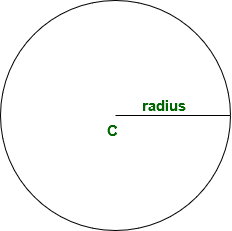C is the centre of circle. Perimeter of a circle is called circumference.

Important properties of a circle:

• Area of circle = π * r * r
• Perimeter of circle = 2 * π * r
or π * diameter

• diameter = 2 * radius

Semicircle:Important properties of a circle:

• Area of semicircle = π * r * r / 2
• Perimeter of semicircle = * π * r
or π * diameter / 2

π is the ratio of circumference to the diameter of circle which is same for all circle or,

```= circumference / diameter
= π or circumference / (2 * radius)
= π ```

π is a non repeating non terminating number which is equal to 22 / 7 or approximately 3.14.

Chord:
It is line segment which connects two points on the circle. Diameter is a special type of chord that passes through centre of circle.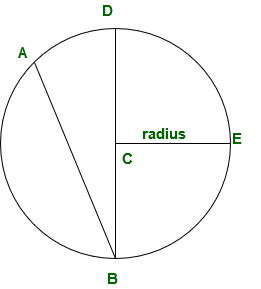AB and BD are chord of given circle.

Arc:
Consider any two point on a circumference of a circle, length between these two pints including all points between them is called arc. Generally an arc contains 3 points to avoid ambiguity.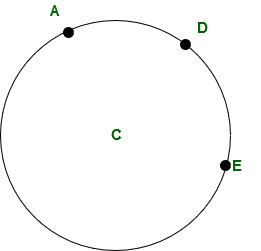ADE is an arc of given circle. D is the point in between A and E to avoid ambiguity.

How to find length of an arc?
Length of the arc = (angle subtend by an arc at centre / 360°)* circumference

What is sector?
Sector is the area covered by an arc.
Area of sector = radius * angle subtends by sector.
∠ ACED = 120°
Area of sector = radius * angle subtends by sector.

Tangent and point of tangency:
A tangent is a line segment which lies on the same plane as a circle and touches the circle at only one point this point is called point of tangency.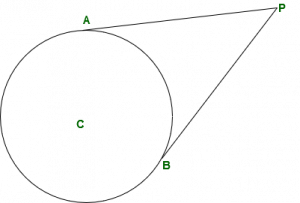AP and BP are tangent of the circle, where A and B are the point of tangency. Radius is drawn to the point of tangency is perpendicular to the tangent.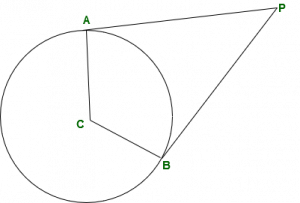Tangent drawn from the same point are equal in length.
or AP = BP.

What is inscribed figure and circumscribed figure?
Inscribed figure: When a circle covers all points of a polygon then the polgon is inscribed in a circle.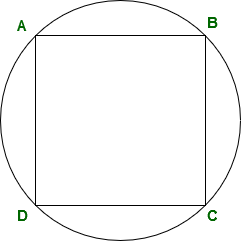Circumscribed figure: When a circle is inside a polygon, its perimeter touches all sides of polygon and every side of polygon is tangent to the circle then the figure is called circumscribed.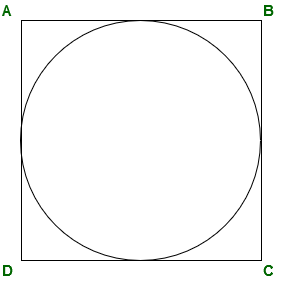What are concetric circles?
Two or more circle with same of different radius are concentric circles.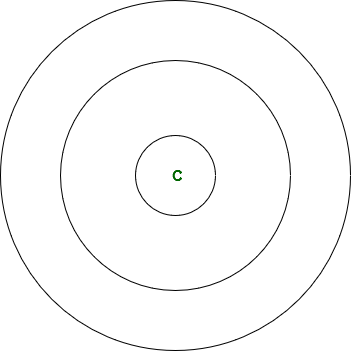Example-1:
What is the radius of circle having area 154 cm2?
Solution:

```Area of circle = π * radius * radius
154 = 22 / 7 * radius * radius (take π = 22 / 7)

Example-2: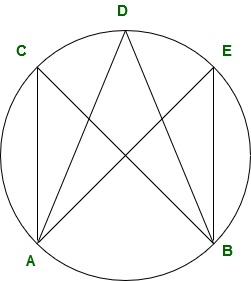If ∠ ADB = 65° then, what is the sum of ∠ ADB, ∠ ACB and ∠ AEB?
Solution:
Angle formed by two points in the same arc are equal.

```∠ ADB = ∠ ACB = ∠ AEB = 65°
∠ ADB + ∠ ACB + ∠ AEB = 195°```

Example-3:
What will be the perimeter of quadrilateral APBC if radius of circle is 5 cm and length of CP is equal to 13?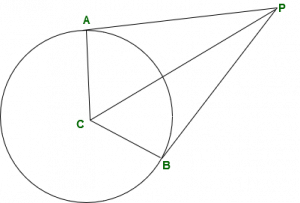Solution:
Angle made by tangent at point of tangency along with radius is always 90°
So, triangle APC is a right angle triangle.
Apply Pythagoras theorem in triangle APC:

```AP2 + AC2 = PC2
AP2 = PC2 - AC2
AP2 = 132 - 52
AP2 = 169 - 25
AP2 = 144
AP = 12 ```

Since tangent from the same external point are equal in length.

```AP = BP = 12 cm

= 12 + 12 + 5 + 5
= 34 cm ```

My Personal Notes arrow_drop_upCheck out this Author's contributed articles.

If you like GeeksforGeeks and would like to contribute, you can also write an article using contribute.geeksforgeeks.org or mail your article to contribute@geeksforgeeks.org. See your article appearing on the GeeksforGeeks main page and help other Geeks.

Please Improve this article if you find anything incorrect by clicking on the "Improve Article" button below.

Article Tags :

Be the First to upvote.

Please write to us at contribute@geeksforgeeks.org to report any issue with the above content.